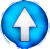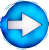The square root of a (necessarily) positive number is always positive: $\sqrt {a^2} = a$ if $a \ge 0$ $\sqrt {a^2} = -a$ if $a \le 0$ $\sqrt {a^2} = \vert a \vert$ (absolute value of a !)Go !

 $\sqrt{(-3)^2} =$ $\vert-3\vert = -(-3) = 3$ $\sqrt{-3}$ doesn't exist, because $-3\lt 0$ $\sqrt{a^4}=$ $\vert a^2 \vert = a^2 \qquad$ because $a^2 \ge 0$